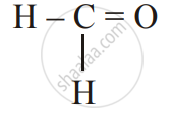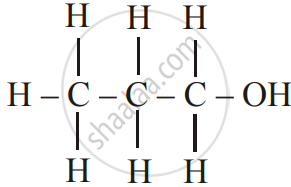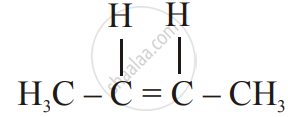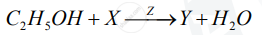# Chemistry Set 1 2017-2018 ICSE Class 10 Question Paper Solution

Chemistry [Set 1]
Date: March 2018

 1
 1.1 | Choose the correct answer from the options given below :
 1.1.1

The salt solution which does not react with ammonium hydroxide is :

Calcium Nitrate

Zinc Nitrate

Copper Nitrate

Concept: Classification of Salts
Chapter: [0.03] Study of Acids, Bases and Salts
 1.1.2

The organic compound which undergoes substitution reaction is :

(A) C_2H_2

(B) C_2H_4

(C) C_10H_18

(D) C_2H_6

Concept: Organic Compounds
Chapter: [0.09] Organic Chemistry
 1.1.3

The electrolysis of acidified water is an example of :

(A) Reduction

(B) Oxidation

(C) Redox reaction

(D) Synthesis

Concept: Electrolytes
Chapter: [0.06] Electrolysis
 1.1.4

The IUPAC name of dimethyl ether is

(A) Ethoxy methane

(B) Methoxy methane

(C) Methoxy ethane

(D) Ethoxy ethane

Concept: Nomenclature of Organic Compounds (IUPAC)
Chapter: [0.09] Organic Chemistry
 1.1.5

The catalyst used in the contact process is

(A) Copper

(B) Iron

(D) Manganese dioxide

Concept: Ethanol
Chapter: [0.09] Organic Chemistry
 1.2 | Give one word or a phase for the following statements :
 1.2.1

The energy released when an electron is added to a neutral gaseous isolated atom to form a negatively charged iron.

Concept: Periodic Properties - Periodic Properties: Electron Affinity
Chapter: [0.01] Periodic Table, Periodic Properties and Variations of Properties
 1.2.2

A process of formation of ions from molecules which are not in the ionic state

Concept: Periodic Properties - Periodic Properties: Ionisation Potential (Ionisation Energy)
Chapter: [0.01] Periodic Table, Periodic Properties and Variations of Properties
 1.2.3

The tendency of an element to form chains of identical atoms.

Concept: Special Features of Carbon
Chapter: [0.09] Organic Chemistry
 1.2.4

The property by which certain hydrated salts, when left exposed to the atmosphere, lose their water of crystallization and crumble into powder.

Concept: Salts
Chapter: [0.03] Study of Acids, Bases and Salts
 1.2.5

The process by which sulphide ore is concentrated.

Concept: Extraction of Metals
Chapter: [0.07] Metallurgy
 1.3
 1.3.1

Write balanced chemical equations to show : The oxidizing action of conc. Sulphuric acid on carbon

Concept: Chemical Properties of Sulphuric Acid
Chapter: [0.084] Sulphuric Acid
 1.3.2

Write a balanced chemical equation for the reaction of sodium hydroxide solution with iron (III) chloride solution

Concept: Acids
Chapter: [0.03] Study of Acids, Bases and Salts
 1.3.3

Write a balanced chemical equation for the action of heat on aluminium hydroxide.

Concept: Types of Elements: Non-metal
Chapter: [0.07] Metallurgy
 1.3.4

Write a balanced chemical equation for the reaction of zinc with potassium hydroxide solution.

Concept: Types of Elements: Non-metal
Chapter: [0.07] Metallurgy
 1.3.5

Write a balanced chemical equation for Action of dilute hydrochloric acid on magnesium sulphite.

Concept: Salts
Chapter: [0.03] Study of Acids, Bases and Salts
 1.4
 1.4.1

Give the IUPAC name for of the following :Concept: Nomenclature of Organic Compounds (IUPAC)
Chapter: [0.09] Organic Chemistry

Give the IUPAC name for of the followingConcept: Nomenclature of Organic Compounds (IUPAC)
Chapter: [0.09] Organic Chemistry

Give the IUPAC name for the followingConcept: Nomenclature of Organic Compounds (IUPAC)
Chapter: [0.09] Organic Chemistry
 1.4.2

Write the structural formula of the two isomers of butane

Concept: Nomenclature of Organic Compounds (IUPAC)
Chapter: [0.09] Organic Chemistry
 1.5
 1.5.1

State one relevant observation for the following.

Lead nitrate solution is treated with sodium hydroxide solution dropwise till it is excess.

Concept: Action of Alkalis on Certain Metals
Chapter: [0.04] Analytical Chemistry
 1.5.2

State one relevant observation for the following:

At the anode, when molten lead bromide is electrolyzed using graphite electrodes.

Concept: Electrolytes
Chapter: [0.06] Electrolysis
 1.5.3

State your observations when Dilute Hydrochloric acid is added to Lead nitrate solution and the mixture is heated.

Concept: General Preparation of Hydrogen Chloride Gas
Chapter: [0.081] Hydrogen Chloride
 1.5.4

State one relevant observation for the following:

Anhydrous calcium chloride is exposed to air for some time.

Concept: Salts
Chapter: [0.03] Study of Acids, Bases and Salts
 1.5.5

State your observations when Barium chloride solution is mixed with Sodium Sulphate Solution.

Concept: Action of Alkalis on Metal Oxides
Chapter: [0.04] Analytical Chemistry
 1.6
 1.6.1

Why do ionic compounds have high melting points

Concept: Electrovalent (or Ionic) Bond
Chapter: [0.02] Chemical Bonding
 1.6.2

Give a reason for Inert gases do not form ions.

Concept: Coordinate Bond
Chapter: [0.02] Chemical Bonding
 1.6.3

Give a reason for Ionisation potential increases across a period, from left to right

Concept: Periodic Properties - Periodic Properties: Ionisation Potential (Ionisation Energy)
Chapter: [0.01] Periodic Table, Periodic Properties and Variations of Properties
 1.6.4

Give a reason for Alkali metals are good reducing agents.

Concept: Types of Elements: Metals
Chapter: [0.07] Metallurgy
 1.6.5

Give a reason for Conductivity of dilute hydrochloric acid is greater than that of acetic acid

Concept: Ethanoic Acid
Chapter: [0.09] Organic Chemistry
 1.7
 1.7.1

Name the gas that is produced in the given cases :

Sulphur is oxidized by concentrated nitric acid.

Concept: Manufacture of Nitric Acid
Chapter: [0.083] Nitric Acid
 1.7.2

Name the gas that is produced in the given cases :

An action of dilute hydrochloride acid on sodium sulphide.

Concept: General Preparation of Hydrogen Chloride Gas
Chapter: [0.081] Hydrogen Chloride
 1.7.3

Name the gas that is produced in the given case:

The action of cold and dilute nitric acid on copper

Concept: Manufacture of Nitric Acid
Chapter: [0.083] Nitric Acid
 1.7.4

Name the gas that is produced in each of the given case :

At the anode during the electrolysis of acidified water.

Concept: Electrolytes
Chapter: [0.06] Electrolysis
 1.7.5

Name the gas that is produced in the given case:

The reaction of ethanol and sodium.

Concept: Types of Elements: Non-metal
Chapter: [0.07] Metallurgy
 1.8 | Fill up the blanks with the correct choice given in brackets.
 1.8.1

Ionic or electrovalent compounds do not conduct electricity in their _______ state. (fused/solid)

Concept: Electrovalent (or Ionic) Bond
Chapter: [0.02] Chemical Bonding
 1.8.2

Electrolysis of aqueous sodium chloride solution will form ________ at the cathode. (Hydrogen gas / Sodium metal)

Concept: Electrolytes
Chapter: [0.06] Electrolysis
 1.8.3

Dry hydrogen chloride gas can be collected by ________ displacement of air. (downward / upward)

Concept: General Preparation of Hydrogen Chloride Gas
Chapter: [0.081] Hydrogen Chloride
 1.8.4

The most common ore of iron is ________. (Calcium / Haematite)

Concept: Types of Separation or Concentration of an Ore
Chapter: [0.07] Metallurgy
 1.8.5

The salt prepared by the method of direct combination is _______. (iron (II) chloride / iron (III) chloride)

Concept: Salts
Chapter: [0.03] Study of Acids, Bases and Salts
 2
 2.1

What do you understand by a lone pair of electrons?

Concept: Electrovalent (or Ionic) Bond
Chapter: [0.02] Chemical Bonding

Draw the electron dot diagram of Hydionium ion (H = 1; O = 8)

Concept: Electrovalent (or Ionic) Bond
Chapter: [0.02] Chemical Bonding
 2.2

In Period 3 of the Periodic Table, element B is placed to the left of element A

On the basis of this information, choose the correct word from the brackets to complete the following statements:

1) The element B would have (lower / higher) metallic character than A

2) The element A would probably have (lesser / higher) electron affinity than B.

3) The element A would have (greater / smaller) atomic size than B.

Concept: Periodic Properties - Periodic Properties: Metallic Character
Chapter: [0.01] Periodic Table, Periodic Properties and Variations of Properties
 2.3

Copy and complete the following table which refers to the conversion of ions to neutral particles.

 Conversion Ionic equation Oxidation / Reduction Chloride ion to chlorine molecule 1)_________ 2)_________ Lead (II) ion to lead 3)_________ 4)_________
Concept: Electrolytes
Chapter: [0.06] Electrolysis
 3
 3.1

Write the balanced chemical equation to prepare ammonia gas in the laboratory by using an alkali.

Concept: Types of Elements: Non-metal
Chapter: [0.07] Metallurgy

State why concentrated sulphuric acid is not used for drying ammonia gas

Concept: Types of Elements: Non-metal
Chapter: [0.07] Metallurgy

Why is ammonia gas not collected over water?

Concept: Types of Elements: Non-metal
Chapter: [0.07] Metallurgy
 3.2

Name the acid used for the preparation of hydrogen chloride gas in the laboratory. Why is this particular acid preferred to other acids?

Concept: Laboratory Preparation of Hydrogen Chloride Gas
Chapter: [0.081] Hydrogen Chloride [0.1] Practical Work

Write the balanced chemical equation for the laboratory preparation of hydrogen chloride gas.

Concept: Laboratory Preparation of Hydrogen Chloride Gas
Chapter: [0.081] Hydrogen Chloride [0.1] Practical Work
 3.3

For the preparation of hydrochloric acid in the laboratory:

Why is the direct absorption of hydrogen chloride gas in water not feasible?

Concept: Laboratory Preparation of Hydrogen Chloride Gas
Chapter: [0.081] Hydrogen Chloride [0.1] Practical Work

For the preparation of hydrochloric acid in the laboratory:

What arrangement is done to dissolve hydrogen chloride gas in water?

Concept: General Preparation of Hydrogen Chloride Gas
Chapter: [0.081] Hydrogen Chloride
 3.4

For the electro-refining of copper: What is the cathode made up of?

Concept: Types of Elements: Non-metal
Chapter: [0.07] Metallurgy

For the electro-refining of copper: Write the reaction that takes place at the anode

Concept: Types of Elements: Non-metal
Chapter: [0.07] Metallurgy
 4
 4.1

The percentage composition of a gas is Nitrogen 82.35%, Hydrogen 17.64%. Find the empirical formula of the gas.  [N = 14, H = 1]

Concept: Mole Concept
Chapter: [0.05] Mole Concept and Stoichiometry
 4.2

Aluminium carbide reacts with water according to the following equation :

Al_4C_3 + 12H_2O-> 4Al(OH)_3 + 3CH_4

1)What mass of aluminium hydroxide is formed from 12g of aluminium carbide?

2) What volume of methane at s.t.p. is obtained from 12g of aluminium carbide?

[Relatively molecular weight of Al_4Cl_3 = 144; Al(OH)_3 = 78]

Concept: Relationship Between Vapour Density and Relative Molecular Mass
Chapter: [0.05] Mole Concept and Stoichiometry
 4.3

If 150 cc of gas A contains X molecules, how many molecules of gas B will be present in 75 cc of B?. The gases A and B are under the same conditions of temperature and pressure.

Concept: Fundamental Laws of Gases - Avogadro’s Law
Chapter: [0.05] Mole Concept and Stoichiometry

Name the law om which same conditions of temperature and pressure

Concept: Fundamental Laws of Gases - Avogadro’s Law
Chapter: [0.05] Mole Concept and Stoichiometry
 4.4

State the main components of the following alloys:

Brass

Concept: Types of Separation or Concentration of an Ore
Chapter: [0.07] Metallurgy

State the main components of the following alloys:

Duralumin

Concept: Types of Separation or Concentration of an Ore
Chapter: [0.07] Metallurgy
 5
 5.1

Complete the following table which relates to the homologous series of hydrocarbons.

 General Formula IUPAC name of the homologous series Characteristic bond type IUPAC name of the first member of the series C_nH_(2n-2) (A)________ (B)______ (C)______ C_nH_(2n+1) (B)________ (E)______ (F)______
Concept: Homologous Series of Carbon Compound
Chapter: [0.09] Organic Chemistry
 5.2

Name the most common ore of the metal aluminium from which the metal is extracted. Write the chemical formula of the ore.

Concept: Types of Separation or Concentration of an Ore
Chapter: [0.07] Metallurgy

Name the process by which impure ore of aluminium gets purified by using the concentrated solution of an alkali.

Concept: Types of Separation or Concentration of an Ore
Chapter: [0.07] Metallurgy

Write the equation for the formation of aluminium at the cathode during the electrolysis of alumina.

Concept: Types of Separation or Concentration of an Ore
Chapter: [0.07] Metallurgy
 6
 6.1

A compound X (having the vinegar-like smell) when treated with ethanol in the presence of the acid Z, gives a compound Y which has a fruity smell.

The reaction is:1) Identify Y and Z.

2) Write the structural formula of X.

3) Name the above reaction.

Concept: Action of Alkalis on Certain Metals
Chapter: [0.04] Analytical Chemistry
 6.2

Ethane burns in oxygen to form CO2 and H2O according to the equation:

2C_2H_6+7O_2 -> 4CO_2 + 6H_2O

If 1250 cc of oxygen is burnt with 300 cc of ethane.

Calculate:

1) the volume of CO_2 formed

2) the volume of unused O_2

Concept: Empirical Formula of a Compound
Chapter: [0.05] Mole Concept and Stoichiometry
 6.3

Three solutions P, Q and R have pH value of 3.5, 5.2 and 12.2 respectively. Which one of these is a:

1) Weak acid?

2) Strong alkali?

Concept: Acids
Chapter: [0.03] Study of Acids, Bases and Salts
 7
 7.1

Give a chemical test to distinguish between the given pairs of chemicals:

Lead nitrate solution and Zinc nitrate solution

Concept: Action of Alkalis on Certain Metals
Chapter: [0.04] Analytical Chemistry

Give a chemical test to distinguish between the given pairs of chemicals:

Sodium chloride solution and Sodium nitrate solution

Concept: General Preparation of Hydrogen Chloride Gas
Chapter: [0.081] Hydrogen Chloride
 7.2

Write a balanced equation for the preparation of the following salts:

Copper sulphate from Copper Carbonate.

Concept: Salts
Chapter: [0.03] Study of Acids, Bases and Salts

Write a balanced equation for the preparation of the following salts:

Zinc carbonate from Zinc sulphate

Concept: Salts
Chapter: [0.03] Study of Acids, Bases and Salts
 7.3

What is the type of salt formed when the reactants are heated at a suitable temperature for the preparation of Nitric acid?

Concept: Laboratory Preparation of Nitric Acid
Chapter: [0.083] Nitric Acid
 7.4

Which property of sulphuric acid is shown by the reaction of the concentrated sulphuric acid with Ethanol?

Concept: Chemical Properties of Sulphuric Acid
Chapter: [0.084] Sulphuric Acid

Which property of sulphuric acid is shown by the reaction of the concentrated sulphuric acid with Carbon?

Concept: Chemical Properties of Sulphuric Acid
Chapter: [0.084] Sulphuric Acid

#### Request Question Paper

If you dont find a question paper, kindly write to us

View All Requests

#### Submit Question Paper

Help us maintain new question papers on Shaalaa.com, so we can continue to help students

only jpg, png and pdf files

## CISCE previous year question papers Class 10 Chemistry with solutions 2017 - 2018

CISCE Class 10 Chemistry question paper solution is key to score more marks in final exams. Students who have used our past year paper solution have significantly improved in speed and boosted their confidence to solve any question in the examination. Our CISCE Class 10 Chemistry question paper 2018 serve as a catalyst to prepare for your Chemistry board examination.
Previous year Question paper for CISCE Class 10 Chemistry-2018 is solved by experts. Solved question papers gives you the chance to check yourself after your mock test.
By referring the question paper Solutions for Chemistry, you can scale your preparation level and work on your weak areas. It will also help the candidates in developing the time-management skills. Practice makes perfect, and there is no better way to practice than to attempt previous year question paper solutions of CISCE Class 10.

How CISCE Class 10 Question Paper solutions Help Students ?
• Question paper solutions for Chemistry will helps students to prepare for exam.
• Question paper with answer will boost students confidence in exam time and also give you an idea About the important questions and topics to be prepared for the board exam.
• For finding solution of question papers no need to refer so multiple sources like textbook or guides.